# Solution September 11, 2007

### Solution

Assume for the sake of contradiction that we have a and b such that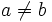$a \neq b$ and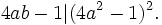$4ab-1 | (4a^2-1)^2.\,$

Then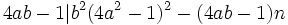$4ab-1 | b^2(4a^2-1)^2 - (4ab-1)n\,$

With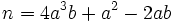$n = 4a^3b + a^2 - 2ab\,$ this gives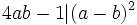$4ab-1 | (a-b)^2\,$

Let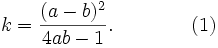$k = \frac{(a-b)^2}{4ab-1}. \qquad\qquad\mbox{(1)}$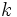$k\,$ can not be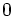$0\,$ so we have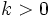$k>0\,$. Choose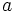$a\,$ and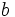$b\,$ among the solutions of (1) such that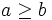$a\geq b\,$ and$a\,$ is minimal.

Then, on the one hand,$k(4ab - 1) = a^2 - 2ab + b^2\,$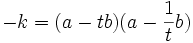$-k = (a - tb)(a - \frac 1t b)$

where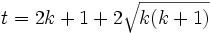$t = 2k+1 + 2\sqrt{k(k+1)}$. Since$a\geq b$, the second term is positive, and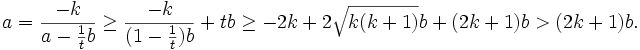$a = \frac{-k}{a - \frac 1t b} \ge \frac{-k}{(1-\frac1t)b} + tb \ge -2k + 2\sqrt{k(k+1)}b + (2k+1)b > (2k+1)b.$

On the other hand, if$a\,$ is a solution of (1) then so is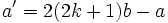$a' = 2(2k+1)b - a\,$. By the minimality condition, we have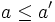$a\leq a'\,$, i.e.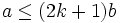$a \leq (2k+1)b\,$

This is a contradiction to the previous inequality, completing the proof.